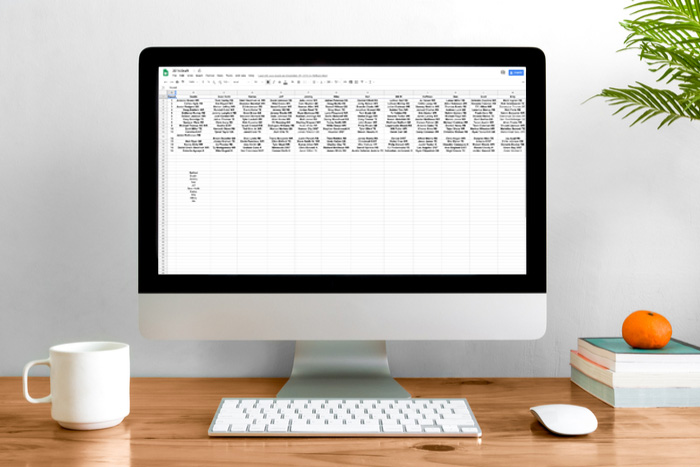How To

# 5 formulas for Google Sheets you didn’t know you neededThe Google Suite has changed the internet in many ways, with its handy features, convenient accessibility, and all-around usefulness. In particular, Google Sheets is a great way to collect and share data with others and to stay organized on the go without having to use Excel. What many may not know is you can find a wide range of formulas that make Google Sheets even more useful. Take a look at five of them below.

## 5 formulas for Google Sheets you didn’t know you needed

### 1. Array formula

The Array formula is a useful one because it can apply formulas in the best possible way to multiple cells. It has a single calculation that funnels results into multiple rows or columns. It’s efficient, and it also avoids the drawback of slowing down Google Sheets when users manually drag formulas across a wide range of cells. To use this formula, use the code ARRAYFORMULA(array_formula).

### 2. Importrange formula

While it may take a very long time to manually move data from one sheet or document to another in Google Sheets, there’s a formula that does all the work for you — and quickly! It will import data from a range of cells from a separate Google Sheet spreadsheet in the blink of an eye. All you have to do is use the following formula: IMPORTRANGE(spreadsheet_url, range_string).

### 3. Find formula

The Find formula is useful for searching for a string of text. To use this, use the formula FIND (search_for, text_to_search, [starting_at]). It’s as easy as that!

### 4. Substitute formula

Want to substitute a new text string in a Google Sheet doc without having to do it manually? It’s simple when you use this formula: SUBSTITUTE(text_to_search, search_for, replace_with, [occurrence_number]).

### 5. Countif formula

This formula is super handy if you have a set of data, and you want to know how many times a single number or block of text is repeated. For example, say you have a spreadsheet where people can RSVP “yes” or “no” to an event. If you want to know how many people said yes, instead of counting by manually, you can just use the formula, COUNTIF, with the range of cells and the value you want it to look for. (Note that if you’re looking for text, it needs to be in quotes in the formula.) It’s a great way to save time, and this way your count should update if one of the values changes.

This is just the beginning of the wide range of useful Google Sheets formulas you have at your fingertips. Start with these and enjoy the productivity boost!